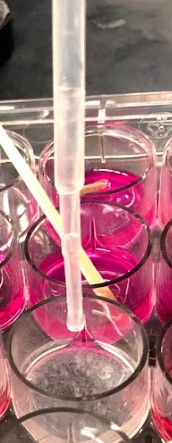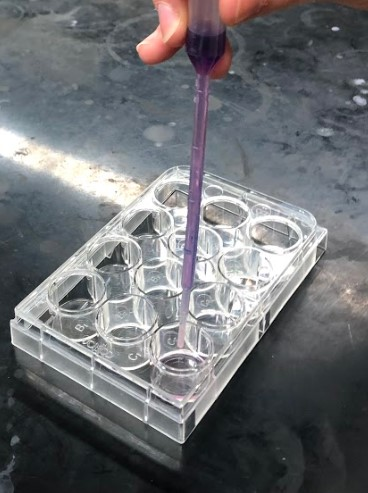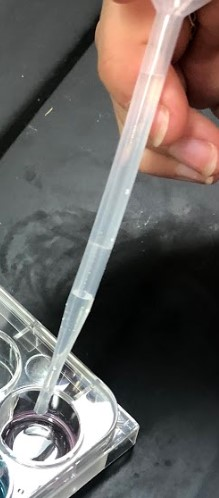# 3: LeChatelier's Principle

$$\newcommand{\vecs}{\overset { \rightharpoonup} {\mathbf{#1}} }$$ $$\newcommand{\vecd}{\overset{-\!-\!\rightharpoonup}{\vphantom{a}\smash {#1}}}$$$$\newcommand{\id}{\mathrm{id}}$$ $$\newcommand{\Span}{\mathrm{span}}$$ $$\newcommand{\kernel}{\mathrm{null}\,}$$ $$\newcommand{\range}{\mathrm{range}\,}$$ $$\newcommand{\RealPart}{\mathrm{Re}}$$ $$\newcommand{\ImaginaryPart}{\mathrm{Im}}$$ $$\newcommand{\Argument}{\mathrm{Arg}}$$ $$\newcommand{\norm}{\| #1 \|}$$ $$\newcommand{\inner}{\langle #1, #2 \rangle}$$ $$\newcommand{\Span}{\mathrm{span}}$$ $$\newcommand{\id}{\mathrm{id}}$$ $$\newcommand{\Span}{\mathrm{span}}$$ $$\newcommand{\kernel}{\mathrm{null}\,}$$ $$\newcommand{\range}{\mathrm{range}\,}$$ $$\newcommand{\RealPart}{\mathrm{Re}}$$ $$\newcommand{\ImaginaryPart}{\mathrm{Im}}$$ $$\newcommand{\Argument}{\mathrm{Arg}}$$ $$\newcommand{\norm}{\| #1 \|}$$ $$\newcommand{\inner}{\langle #1, #2 \rangle}$$ $$\newcommand{\Span}{\mathrm{span}}$$$$\newcommand{\AA}{\unicode[.8,0]{x212B}}$$

Objectives

• To perturb chemical reactions at equilibrium and observe how they respond.
• To explain these observations using Le Chatelier’s Principle.

All chemical reactions eventually reach a state in which the rate of the reaction in the forward direction is equal to the rate of the reaction in the reverse direction. When a reaction reaches this state, it is said to be at chemical equilibrium. The concentrations of reactants and products at equilibrium are constant as a function of time. Thus, for a homogeneous aqueous system of the form

$a\text{A} (aq) + b\text{B} (aq) \ce{<=>}c\text{C} (aq) + d\text{D} (aq) \label{1}$

we can express the equilibrium-constant expression for this reaction as,

$K_{c}= \frac{[\text{C}]^{c}[\text{D}]^{d}}{[\text{A}]^{a}[\text{B}]^{b}} \label{2}$

where the values of $$[\text{A}]$$, $$[\text{B}]$$, $$[\text{C}]$$, and $$[\text{D}]$$ correspond to the equilibrium concentrations (or equilibrium positions) of all the aqueous chemical components, and $$a$$, $$b$$, $$c$$, and $$d$$ are their respective stoichiometric coefficients. Note that for a heterogeneous system including pure solids or liquids of the form

$a\text{A} (aq) + b\text{B} (s) \ce{<=>}c\text{C} (aq) + d\text{D} (l) \label{3}$

the pure liquids and solids do not appear in the equilibrium-constant expression:

$K_{c}= \frac{[\text{C}]^{c}}{[\text{A}]^{a}} \label{4}$

It has been observed that when a reaction at equilibrium is perturbed by applying a stress, the reaction will respond by shifting its equilibrium position so as to counteract the effect of the perturbation/stress. In other words, the concentrations of the reactants and products will shift so that the relationship described by Equation \ref{2} is again satisfied. This idea was first proposed by Henri-Louis Le Chatelier and has since been referred to as, “Le Chatelier’s Principle”.

For chemical reactions at equilibrium in aqueous solution, the most common types of perturbations include changing the concentration of one of the aqueous solutes, changing the concentrations of all aqueous solutes by changing the total solution volume, or changing the temperature. The general responses of an aqueous system to these particular perturbations are tabulated below.

Perturbation Effect on Equilibrium Position Effect on $$K_{c}$$
Increase in concentration of a single reactant, or, decrease in concentration of a single product. Shift to the right None
Decrease in concentration of a single reactant, or, increase in concentration of a single product. Shift to the left None
Decrease in all aqueous concentrations due to an increase in solution volume resulting from the addition of solvent Shift towards side with more solute particles None
Increase in all aqueous concentrations due to a decrease in solution volume resulting from the removal of solvent (evaporation) Shift towards side with less solute particles None
Increase temperature of an exothermic reaction Shift to the left Decrease
Decrease temperature of an exothermic reaction Shift to the right Increase
Increase temperature of an endothermic reaction Shift to the right Increase
Decrease temperature of an endothermic reaction Shift to the left Decrease
Addition of an inert substance, catalyst, pure liquid, or pure solid None None

Notice that only a temperature change can affect the value of $$K_{c}$$; in all other cases the value of $$K_{c}$$ remains constant.

In this experiment you will perturb reactions that have attained equilibrium. You will then observe how each reaction responds to that perturbation in order to restore equilibrium. In your report you describe these changes in terms of Le Chatelier’s Principle.

## Procedure

Safety And Waste Disposal

• The solutions in part I and II are able to be disposed of down the drain.
• The solutions in part III need to be collected in the waste container.
• Extreme caution should be exercised when using concentrated hydrochloric acid.

### Part A: Acid-Base Equilibrium

$\ce{HSO^{-}_{4}} (aq) + \ce{H_{2}O} (l) \ce{<=>} \ce{H_{3}O^{+}} (aq) + \ce{SO^{2-}_{4}} (aq) \label{5}$

###### Step 1

Clean and dry a well plate.

###### Step 2Using a clean and dry plastic pipette, add 10 drops of $$\ce{NaHSO_{4}}$$ to wells A1, A2, and A3. Add 20 drops of the solution to wells A4.

###### Step 3Add 1 drop of thymol blue indicator solution from a dropper bottle to the $$\ce{NaHSO_{4}}$$ solution in each well. Thoroughly stir each mixture with a clean toothpick. Observe and record the color of the mixture in each well. They should all be the same color at this point.

###### Step 4Using a clean and dry plastic pipette, add 3 drop of the $$\ce{Na_{2}SO_{4}}$$ solution to the mixture in well A1. Thoroughly mix the solution with a clean toothpick, and compare the color of the mixture with that of the standard in well A2. Continue to add drops of the $$\ce{Na_{2}SO_{4}}$$ solution to well A1 until you see a color change, stirring after each addition. Record your observations.

###### Step 5Add a crystal of solid $$\ce{NaHSO_{4}}$$ to well A3. Stir the mixture with a clean toothpick to dissolve the crystal. Continue adding individual crystals of $$\ce{NaHSO_{4}}$$ until you see a color change. Record your observations.

###### Step 6Draw the entire mixture in well A4 into one of the rinsed plastic pipettes. Carefully invert the pipette, allowing the solution to fill the pipette bulb. Hold the inverted pipette tip and tap the bulb on the counter to drain the solution from the stem of the pipette into the bulb. Place this pipette, bulb down, in the hot water bath. Occasionally stir the pipette and contents in the hot water bath to speed the heating process. Observe and record any color change of the mixture in the pipette.

###### Step 7

Discard the solutions down the drain.

### Part B: Dissolving Insoluble Solids

$\ce{Mg(OH)_{2}} (s) \ce{<=>} \ce{Mg^{2+}} (aq) + \ce{OH^{-}} (aq) \label{6}$

###### Step 1Using a clean and dry plastic pipette, add 20 drops of 1.0M $$\ce{MgCl_{2}}$$ solution to wells B1, B2, B3, and B4.

###### Step 2Add 1 drop of phenolphthalein indicator solution from a dropper bottle to the $$\ce{MgCl_{2}}$$ solution in wells B3 and B4. Thoroughly stir each mixture with a clean toothpick, leaving the toothpicks in the wells.

###### Step 3Using a clean and dry plastic pipette, add 10 drops of $$\ce{NaOH}$$ solution to the $$\ce{MgCl_{2}}$$ solution in wells B1, B2, B3, and B4. Thoroughly mix the solution with toothpicks and leave the toothpicks in the wells. Record your observations, carefully noting the appearance and color of the mixtures and the presence or absence of a precipitate.

###### Step 4Add 1 drop of concentrated $$\ce{HCl}$$ solution from the dropper bottle to the mixture in well B1 and stir. Continue adding the $$\ce{HCl}$$ solution drop wise until you see a change. Record your observations.

###### Step 5Add 1 drop of $$\ce{Na_{4}EDTA}$$ solution from the dropper bottle to the mixture in well B2, and stir. Continue adding the $$\ce{Na_{4}EDTA}$$ solution drop wise until you see a change. Record your observations.

###### Step 6Draw the entire mixture in well B3 into the other rinsed plastic pipette. Carefully invert the pipette, allowing the solution to fill the pipette bulb. Hold the inverted pipette tip and tap the bulb on the counter to drain the solution from the stem of the pipette into the bulb. Place this pipette, bulb down, in the ice water bath. Occasionally stir the pipette and contents in the ice water bath to speed the cooling process. Allow the pipette to remain in the water bath for 3 - 4 minutes. Observe and record any color change of the mixture in the pipette comparing it to well B4.

###### Step 7

Discard the solutions down the drain.

### Part C: Complex Ion Equilibrium

$\ce{CoCl^{2-}_{4}} (MeOH) + 6\ce{H_{2}O} (l) \ce{<=>} \ce{Co(H_{2}O)_{6}} (aq) + 4\ce{Cl^{-}} (aq) \label{7}$

###### Step 1Using a clean and dry plastic pipette, add 15 drops of 0.15 M $$\ce{CoCl^{2-}_{4}}$$ $$\ce{MeOH}$$ solution to wells C1-C7. Make sure the well plate is completely dry before adding the solution.

###### Step 2Using a clean plastic pipette, add just enough water to the purple/blue solution to wells C2 to change the color. Thoroughly mix the solution with toothpick and leave the toothpicks in the wells. Record your observations. C1 will serve as your pre water addition comparison and C2 will serve as your post water addition comparison.

###### Step 3Add solid crystals of $$\ce{CaCl_{2}}$$ to well C3 and stir. Continue adding crystals until you notice a change. Record your observations.

###### Step 4Add 1 drop of concentrated $$\ce{HCl}$$ solution from the dropper bottle to the mixture in well C4 and stir. Continue adding the $$\ce{HCl}$$ solution drop wise until you see a change. Record your observations.

###### Step 5Add 1 drop of 0.1 M $$\ce{AgNO_{3}}$$ solution from the dropper bottle to the mixture in well C5 and stir. Continue adding the $$\ce{AgNO_{3}}$$ solution drop wise until you see a change. Record your observations.

###### Step 6Draw the entire mixture in well C6 into a dry plastic pipette. Carefully invert the pipette, allowing the solution to fill the pipette bulb. Hold the inverted pipette tip and tap the bulb on the counter to drain the solution from the stem of the pipette into the bulb. Place the pipette, bulb down, in the hot water bath. Occasionally stir the pipette and contents in the hot water bath to speed the heating process. Allow the pipette to remain in the water bath for 3 - 4 minutes or until you see a change. Observe and record any color change of the mixture in the pipette comparing it to well C1 and C2. Repeat using C7 and the ice bath. Do your results agree with one another? Record your results.

###### Step 7

Discard the solutions in the waste container.

### Part A – Equilibrium and an Acid-Base Indicator

Equilibrium system: $$\ce{HSO^{-}_{4}} (aq) + \ce{H_{2}O} (l) \ce{<=>} \ce{H_{3}O^{+}} (aq) + \ce{SO^{2-}_{4}} (aq)$$

Observations

Record your results upon completing each of the following steps:

### Part B – Solubility Equilibrium and $$K_{sp}$$

Equilibrium system: $$\ce{Mg(OH)_{2}} (s) \ce{<=>} \ce{Mg^{2+}} (aq) + \ce{OH^{-}} (aq)$$

Observations

### Part C – Complex Ion Equilibria

Equilibrium system: $$\ce{CoCl^{2-}_{4}} (MeOH) + 6\ce{H_{2}O} (l) \ce{<=>} \ce{Co(H_{2}O)_{6}} (aq) + 4\ce{Cl^{-}} (aq)$$

Observations

3: LeChatelier's Principle is shared under a not declared license and was authored, remixed, and/or curated by LibreTexts.